# POLY2MASK and ROIPOLY – Part 3

This is the final post in a series discussing the algorithm underlying Image Processing Toolbox functions poly2mask and roipoly. (See Part 1 and Part 2.)

There are several desirable properties for a polygon scan conversion algorithm to have. It helps to visualize some of these properties if you look first at a set of polygons that completely covers the image region, with no gaps and no overlaps.

imshow(ones(20,20), 'InitialMag', 'fit');
hold on
for x = 0.5:20.5
for y = 0.5:20.5
plot([x x], [0.5 20.5], 'Color', [.8 .8 .8]);
plot([0.5 20.5], [y y], 'Color', [.8 .8 .8]);
end
end

x1 = [0.5 10 0.5 0.5];
y1 = [0.5 0.5 20.5 0.5];

x2 = [10 20.5 20.5 0.5 10];
y2 = [0.5 0.5 4 20.5 0.5];

x3 = [20.5 20.5 0.5 20.5];
y3 = [4 20.5 20.5 4];

plot(x1, y1, 'Color', 'r', 'LineWidth', 3, 'Clipping', 'off');
plot(x2, y2, 'Color', 'r', 'LineWidth', 3, 'Clipping', 'off');
plot(x3, y3, 'Color', 'r', 'LineWidth', 3, 'Clipping', 'off');

text(4, 4, 'Polygon 1', 'HorizontalAlignment', 'center')
text(12, 7, 'Polygon 2', 'HorizontalAlignment', 'center')
text(16, 16, 'Polygon 3', 'HorizontalAlignment', 'center')
hold off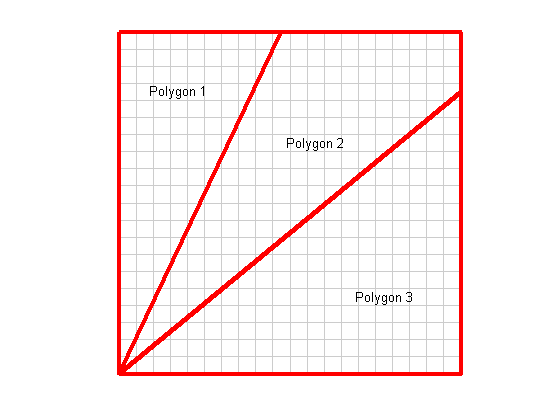Here's what we would like to achieve from our polygon scan conversion:

• There should be no gaps. That is, since the three polygons cover the entire image, every pixel should be assigned to at least one polygon.
• There should be no overlaps. That is, since the three polygons do not overlap in area, no pixel should be assigned to more than polygon.
• Pixel assignment to the three polygons should be insensitive to vertex ordering. It should make no difference which polygon vertex is listed first, or whether the vertices are listed in clockwise or counterclockwise order.
• Pixel assignment to the three polygons should be unaffected by floating-point round-off.

So what rule should we use to decide how to assign pixels that are partially inside and outside of a polygon? One possible rule is that a pixel is considered to be inside a polygon if it is partially inside the polygon. But that violates the no-overlap rule, since a pixel can be partially inside more than one polygon.

Another possible rule is that a pixel is considered to be inside a polygon if at least half of the pixel (by area) is inside the polygon. That violates the no-gap rule, though. Consider the lower-left corner of the image above:

axis([0.5 2.5 18.5 20.5])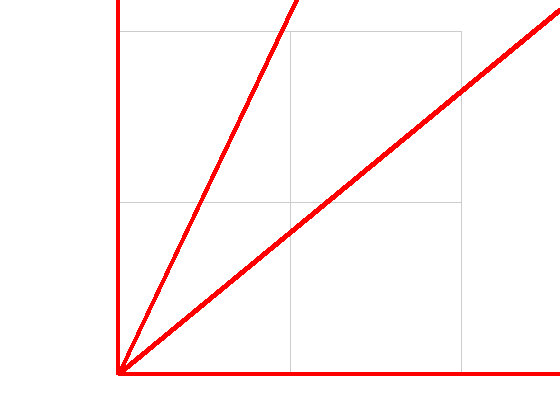None of the three polygons covers more than half of the lower-left pixel.

The rule used by poly2mask is that a pixel is inside a polygon if and only if its center is inside the polygon.

Now we have to consider how to deal cleanly with all the special cases I mentioned in Part 2. Basically, trouble happens whenever a ray passes directly through one or more vertices, or along an edge. poly2mask basically avoids these problems entirely by approximating the polygon by tiny horizontal and vertical line segments that lie on the edges of a 5-by-5 subpixel grid. It looks like this:

clf
hold on
for x = linspace(0.5,1.5,6)
for y = linspace(0.5,1.5,6)
plot([x x], [0.5 1.5], 'Color', [.8 .8 .8]);
plot([0.5 1.5], [y y], 'Color', [.8 .8 .8]);
end
end
plot([0.5 1.5], [0.5 1.5], 'b', 'LineWidth', 2)
axis equal
axis ij
plot([0.5 0.5 0.7 0.7 0.9 0.9 1.1 1.1 1.3 1.3 1.5], ...
[0.5 0.7 0.7 0.9 0.9 1.1 1.1 1.3 1.3 1.5 1.5], ...
'r', 'LineWidth', 2, 'Clipping', 'off')
plot(1.0, 1.0, '*')
hold off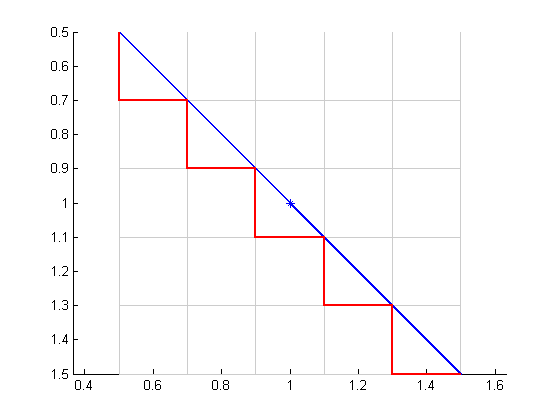The plot above shows a single pixel. The blue line represents an original edge of the polygon. The red line approximates the blue line using horizontal and vertical segments that lie on the gray grid.

Notice how none of the edges or vertices of the approximation can ever intersect the center of the pixel. Therefore, if we follow rays along pixel centers, we will never encounter vertices of the modified polygon, nor will any ray be coincident with an edge. That way, the algorithm can be coded with no special cases.

x = [10 35 40 10];
y = [10 40 15 10];
vispoly2mask(x,y,50,50)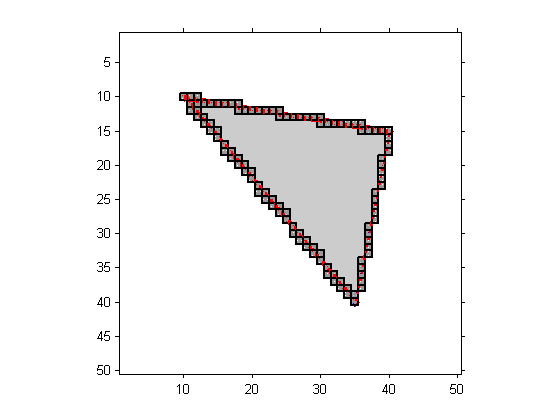We need to zoom into a corner of the polygon to see more detail.

axis([8 15 8 15])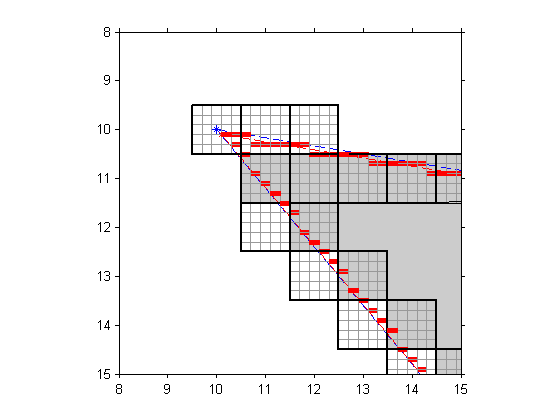The red bars are the horizontal edges of the polygon approximation. poly2mask scans down the center of each image column, keeping track of the number of edge crossings, and using the even-odd rule to decide whether each pixel is inside the polygon.

Here's the final result displayed as a binary image with the polygon superimposed.

bw = poly2mask(x,y,50,50);
imshow(bw, 'InitialMag', 'fit')
hold on
plot(x,y,'r','LineWidth',2)
hold off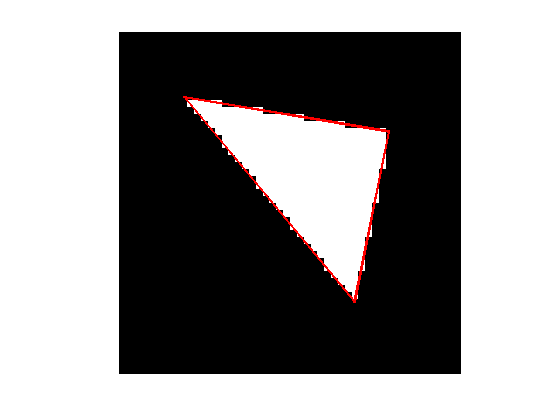Published with MATLAB® 7.3

|

### コメント

コメントを残すには、ここ をクリックして MathWorks アカウントにサインインするか新しい MathWorks アカウントを作成します。